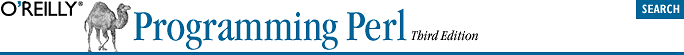home | O'Reilly's CD bookshelfs | FreeBSD | Linux | Cisco | Cisco Exam## 3.7. Multiplicative Operators

Perl provides the C-like operators * (multiply), / (divide), and % (modulo). The * and / work exactly as you would expect, multiplying or dividing their two operands. Division is done in floating point, unless you've used the integer pragmatic module.

The % operator converts its operands to integers before finding the remainder according to integer division. (However, it does this integer division in floating point if necessary, so your operands can be up to 15 digits long on most 32-bit machines.) Assume that your two operands are called \$a and \$b. If \$b is positive, then the result of \$a % \$b is \$a minus the largest multiple of \$b that is not greater than \$a (which means the result will always be in the range 0 .. \$b-1). If \$b is negative, then the result of \$a % \$b is \$a minus the smallest multiple of \$b that is not less than \$a (which means the result will be in the range \$b+1 .. 0).

When use integer is in scope, % gives you direct access to the modulus operator as implemented by your C compiler. This operator is not well defined for negative operands, but will execute faster.

Binary x is the repetition operator. Actually, it's two operators. In scalar context, it returns a concatenated string consisting of the left operand repeated the number of times specified by the right operand. (For backward compatibility, it also does this in list context if the left argument is not in parentheses.)

```print '-' x 80;                             # print row of dashes
print "\t" x (\$tab/8), ' ' x (\$tab%8);      # tab over```
In list context, if the left operand is a list in parentheses, the x works as a list replicator rather than a string replicator. This is useful for initializing all the elements of an array of indeterminate length to the same value:
```@ones = (1) x 80;           # a list of 80 1's
@ones = (5) x @ones;        # set all elements to 5```
Similarly, you can also use x to initialize array and hash slices:
```@keys = qw(perls before swine);
@hash{@keys} = ("") x @keys;```
If this mystifies you, note that @keys is being used both as a list on the left side of the assignment and as a scalar value (returning the array length) on the right side of the assignment. The previous example has the same effect on %hash as:
```\$hash{perls}  = "";
\$hash{before} = "";
\$hash{swine}  = "";```